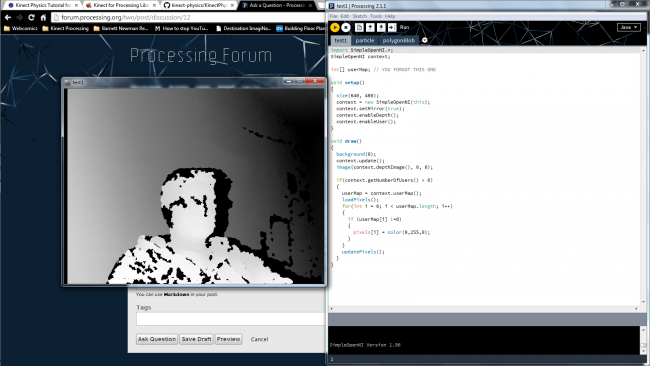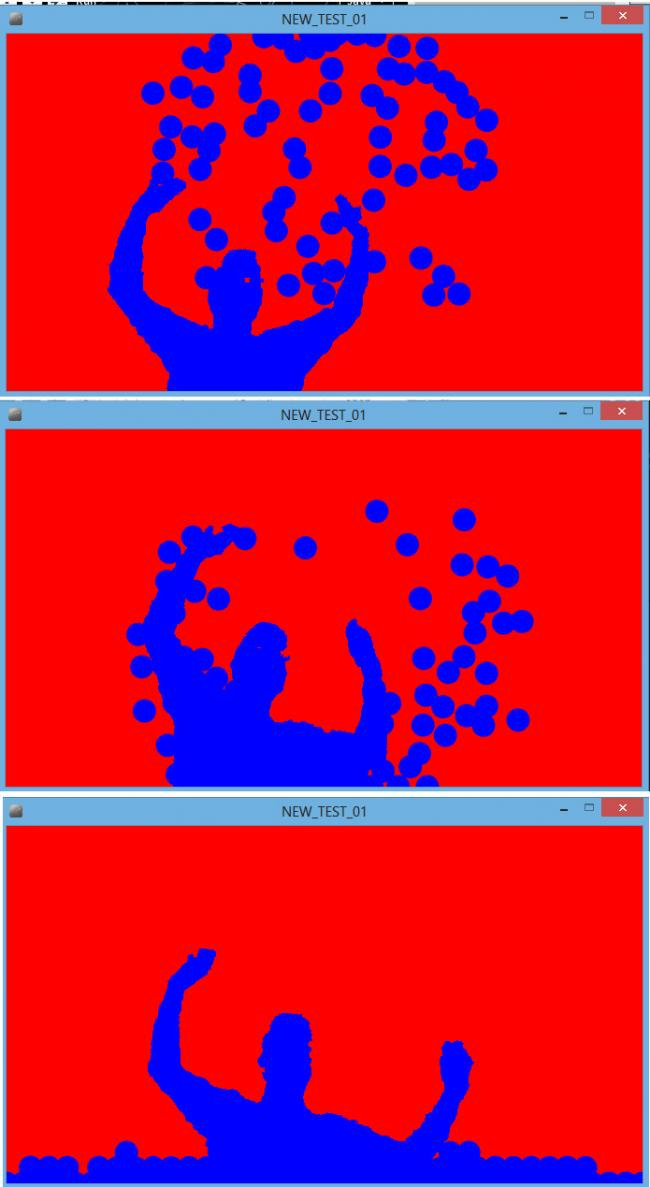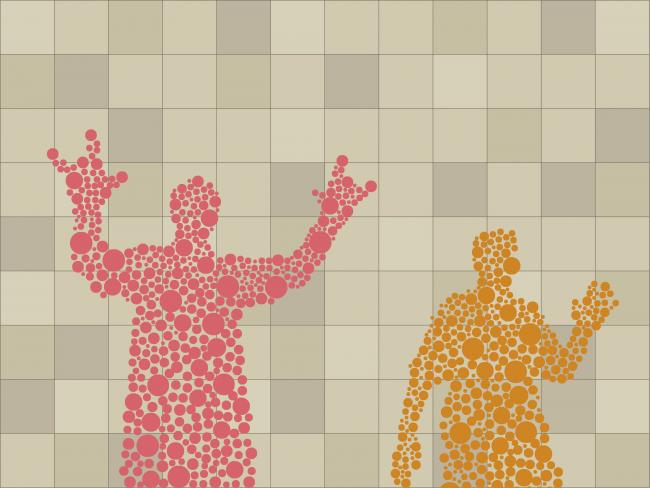#### Howdy, Stranger!

We are about to switch to a new forum software. Until then we have removed the registration on this forum.

# Kinect Silhouettes Just Won't Work

edited February 2014 in Kinect

Hey all

I'm very new to Processing, and I've been going insane trying to get a simple tutorial (the first one on this page: http://www.creativeapplications.net/processing/kinect-physics-tutorial-for-processing/) to work correctly. The Kinect runs fine, but instead of the colored outlines, all I get is a splotchy white shape of me.I've tried just about every tutorial I can find (and not just about silhouettes), but they just don't run. I'm running Processing 2.1.1, OpenSimpleNI 1.96, Windows 7 Professional 64-bit.

Here's the code I've got so far (it's a little different from the code on the link above, because the language has changed a bit since that tutorial was posted):

``````import SimpleOpenNI.*;
SimpleOpenNI context;

int[] userMap;

void setup()
{
size(640, 480);
context = new SimpleOpenNI(this);
context.setMirror(true);
context.enableDepth();
context.enableUser();
}

void draw()
{
background(0);
context.update();
image(context.depthImage(), 0, 0);

if(context.getNumberOfUsers() > 0)
{
userMap = context.userMap();
for(int i = 0; i < userMap.length; i++)
{
if (userMap[i] !=0)
{
pixels[i] = color(0,255,0);
}
}
updatePixels();
}
}
``````

If anyone can help me, that would be great!

Tagged:

• hi,

try out this code in draw method.

``````void draw(){

// background(255,255,255);
context.update();
rgbImage=context.rgbImage();

userMap=context.userMap();
for(int y=0;y<context.depthHeight();y++){
for(int x=0;x<context.depthWidth();x++){
int index=x+y*640;
if(userMap[index]!=0){
pixelColor=rgbImage.pixels[index];
userImage.pixels[index]=color(0,255,0);
}else{
userImage.pixels[index]=color(0);
}

}
}
userImage.updatePixels();
image(userImage,0,0);
}
``````
• Nuts, sorry, I'm new on this forum; didn't realize "accept" meant "mark as correct."

Anyway, I tried running your code--thanks, by the way--with a couple modifications ("pixelColor" had to be initialized as a color variable, "userImage" and "rgbImage" hadn't been initialized at all), but then it started throwing Null Pointer Exceptions in line 35 (16 in your example).

This one: userImage.pixels[index] = color(0, 0, 0);

Is this because we're trying to initialize userImage multiple times?

• hi, M so sorry, i forgot to mention about userImage.

You need to initialize the userImage in setup method, by `userImage=createImage(width,height,RGB);` & we have already initialize rgbImage in draw method.

• I just tried both of your codes (and the one from the link) and none of them work. When I tried your draw function, Archana, the console flashed nullPointerException, then went blank. The sketch window never initializes and Java crashes when I try to close out. I have Processing 2.1.1 and OpenNI 1.96 as well, but I'm on Win 8.1 x64

• Hi Roch,

You have to declare the DepthMap and rgb generation to be enabled in setup method.

Here is the sample code:

``````import SimpleOpenNI.*;
SimpleOpenNI context;
PImage userImage;
int[] userMap;
PImage rgbImage;
color pixelColor;
void setup(){

size(640,480);
context=new SimpleOpenNI(this);
context.enableRGB();
context.enableDepth();
context.enableUser();

userImage=createImage(640,480,RGB);
}
void draw(){

background(0);
context.update();
rgbImage=context.rgbImage();

userMap=context.userMap();
for(int y=0;y<context.depthHeight();y++){
for(int x=0;x<context.depthWidth();x++){
int index=x+y*640;
if(userMap[index]!=0){
pixelColor=rgbImage.pixels[index];
userImage.pixels[index]=color(0,255,0);
}else{
userImage.pixels[index]=color(0);
}

}
}
userImage.updatePixels();
image(userImage,0,0);
}
``````

I am working with Processing-1.5.1, SimpleOpenNI 1.96 on Windows 7 64 bit OS.

• That's very strange. I have the exact same thing but it doesn't run, but when I copy and paste your code, it works fine (in 1.5.1, it stalls out in 2.1.1) Here is what I had:

``````import SimpleOpenNI.*;
SimpleOpenNI context;

int[] userMap;
PImage rgbImage;
PImage userImage;
color pixelColor;

void setup() {
size(640, 480);
context = new SimpleOpenNI(this);
context.enableRGB();
context.enableDepth();
context.enableUser();
userImage = createImage(width, height, RGB);
}

void draw() {
background(0);

context.update();
rgbImage=context.rgbImage();

userMap=context.userMap();
for(int y=0;y<context.depthHeight();y++){
for(int x=0;x<context.depthWidth();x++){
int index=x+y*640;
if(userMap[index]!=0){
pixelColor=rgbImage.pixels[index];
userImage.pixels[index]=color(0,255,0);
}else{
userImage.pixels[index]=color(0);
}

}
}
userImage.updatePixels();
image(userImage,0,0);
}
``````
• I also tried the Kinect Physics Tutorial but had similar problems as Anim9. I am now trying to intergrate the code pasted above by Archana11 with an example (Attraction2D)from the verletphysics library. This is what we are getting: }What I am trying to do is use the silhouette as attraction point rather than the mouse click (as in the original Attraction2D code) and hoping the outcome would be something like the concept image attached:Have attached my code below, can't understand how to make the silhouette the attractor. Any help would be much appreciated. Thanks!

I am using Processing 1.5.1 & 2.1.1, Windows 8 64bit.

``````import toxi.geom.*;
import toxi.physics2d.*;
import toxi.physics2d.behaviors.*;
import SimpleOpenNI.*;
SimpleOpenNI context;
PImage userImage;
int[] userMap;
PImage rgbImage;
color pixelColor;

int NUM_PARTICLES = 75;

VerletPhysics2D physics;
AttractionBehavior mouseAttractor;

//Vec2D uImage;
Vec2D mousePos;

void setup() {
size(680, 382,P3D);
context=new SimpleOpenNI(this);
context.enableRGB();
context.enableDepth();
context.enableUser();
// setup physics with 10% drag
physics = new VerletPhysics2D();
physics.setDrag(0.05f);
physics.setWorldBounds(new Rect(0, 0, width, height));
// the NEW way to add gravity to the simulation, using behaviors
userImage=createImage(640,480,RGB);
}

VerletParticle2D p = new VerletParticle2D(Vec2D.randomVector().scale(5).addSelf(width / 2, 0));
// add a negative attraction force field around the new particle
}

void draw() {
background(255,0,0);
noStroke();
fill(0,0,255);
context.update();
rgbImage=context.rgbImage();

userMap=context.userMap();
for(int y=0;y<context.depthHeight();y++){
for(int x=0;x<context.depthWidth();x++){
int index=x+y*640;
if(userMap[index]!=0){
pixelColor=rgbImage.pixels[index];
userImage.pixels[index]=color(0,0,255);
}else{
userImage.pixels[index]=color(255,0,0);
}

}
}
userImage.updatePixels();
image(userImage,0,0);
if (physics.particles.size() < NUM_PARTICLES) {
}
physics.update();
for (VerletParticle2D p : physics.particles) {
ellipse(p.x, p.y, 25, 25);
}
}

{
// userMap = new Vec2D(image(userImage);
// create a new positive attraction force field around the mouse position (radius=250px)
//UserAttractor = new AttractionBehavior(PImage, 250, 0.9f);
}

void mousePressed() {
mousePos = new Vec2D(mouseX, mouseY);
// create a new positive attraction force field around the mouse position (radius=250px)
mouseAttractor = new AttractionBehavior(mousePos, 250, 0.9f);
}

void mouseDragged() {
// update mouse attraction focal point
mousePos.set(mouseX, mouseY);
}

void mouseReleased() {
// remove the mouse attraction when button has been released
physics.removeBehavior(mouseAttractor);
``````

Has anyone managed to make the other 2 examples work, on the creativeapplication.net link posted above by Anim9?

• Hi,

May be, but if you are trying to get the user's center mass, and then try to attract particles from their positions...

• Any luck with this? Having the same problem, and nobody seems to solve it.. :( @abdoak - I am unable to run your last code posted.. Is it incomplete?

• Hey can we use http://www.lab-eds.org/punktiert - punktiert Library for this stuff .... ??? :-/

I have run this library's example and I think it can be useful to make more interesting stuff with kinect...

Any suggestions .. ???